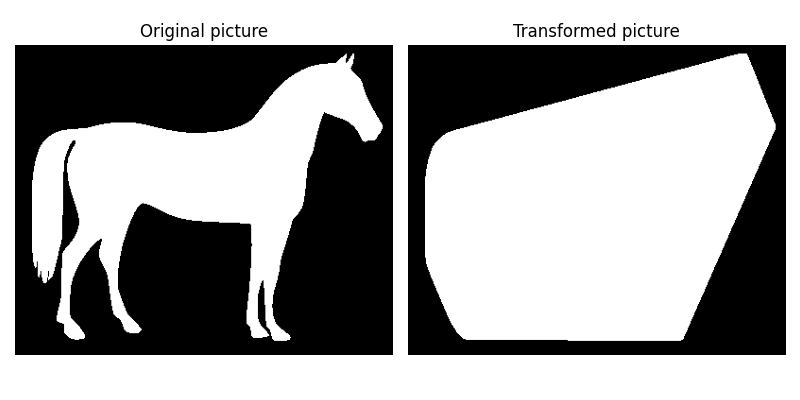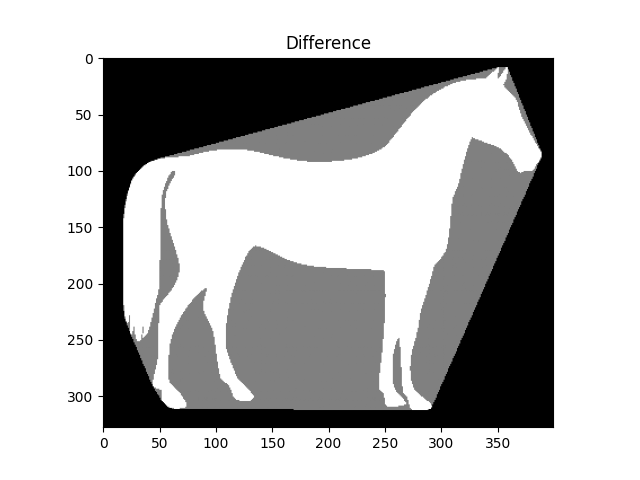# Convex Hull#

The convex hull of a binary image is the set of pixels included in the smallest convex polygon that surround all white pixels in the input.

A good overview of the algorithm is given on Steve Eddin’s blog.

```import matplotlib.pyplot as plt

from skimage.morphology import convex_hull_image
from skimage import data, img_as_float
from skimage.util import invert

# The original image is inverted as the object must be white.
image = invert(data.horse())

chull = convex_hull_image(image)

fig, axes = plt.subplots(1, 2, figsize=(8, 4))
ax = axes.ravel()

ax.set_title('Original picture')
ax.imshow(image, cmap=plt.cm.gray)
ax.set_axis_off()

ax.set_title('Transformed picture')
ax.imshow(chull, cmap=plt.cm.gray)
ax.set_axis_off()

plt.tight_layout()
plt.show()
```We prepare a second plot to show the difference.

```chull_diff = img_as_float(chull.copy())
chull_diff[image] = 2

fig, ax = plt.subplots()
ax.imshow(chull_diff, cmap=plt.cm.gray)
ax.set_title('Difference')
plt.show()
```Total running time of the script: ( 0 minutes 0.418 seconds)

Gallery generated by Sphinx-Gallery